Test: Engineering Mathematics- 9

# Test: Engineering Mathematics- 9

Test Description

## 10 Questions MCQ Test RRB JE for Computer Science Engineering | Test: Engineering Mathematics- 9

Test: Engineering Mathematics- 9 for Computer Science Engineering (CSE) 2022 is part of RRB JE for Computer Science Engineering preparation. The Test: Engineering Mathematics- 9 questions and answers have been prepared according to the Computer Science Engineering (CSE) exam syllabus.The Test: Engineering Mathematics- 9 MCQs are made for Computer Science Engineering (CSE) 2022 Exam. Find important definitions, questions, notes, meanings, examples, exercises, MCQs and online tests for Test: Engineering Mathematics- 9 below.
Solutions of Test: Engineering Mathematics- 9 questions in English are available as part of our RRB JE for Computer Science Engineering for Computer Science Engineering (CSE) & Test: Engineering Mathematics- 9 solutions in Hindi for RRB JE for Computer Science Engineering course. Download more important topics, notes, lectures and mock test series for Computer Science Engineering (CSE) Exam by signing up for free. Attempt Test: Engineering Mathematics- 9 | 10 questions in 30 minutes | Mock test for Computer Science Engineering (CSE) preparation | Free important questions MCQ to study RRB JE for Computer Science Engineering for Computer Science Engineering (CSE) Exam | Download free PDF with solutions
 1 Crore+ students have signed up on EduRev. Have you?
Test: Engineering Mathematics- 9 - Question 1

### A sample of 15 data is as follows: 17, 18, 17, 17, 13, 18, 5, 5, 6, 7, 8, 9, 20, 17, 3. the mode of the data is

Detailed Solution for Test: Engineering Mathematics- 9 - Question 1

Mode refers the most frequently appeared value (which is 17 in this case).

Test: Engineering Mathematics- 9 - Question 2

### In a Poisson distribution if 2P(X = 1) = P(X = 2), then what is the mean?

Detailed Solution for Test: Engineering Mathematics- 9 - Question 2

If X is random variable, then Poisson’s distribution is given by: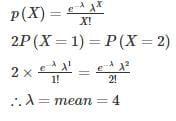Test: Engineering Mathematics- 9 - Question 3

### The continuous distribution variable X is distributed uniformly where X ~ U(6,12). Find the mean of X?

Detailed Solution for Test: Engineering Mathematics- 9 - Question 3

X ~ U(6,12)

Comparing with X ~ U(a,b)

∴ a = 6 and b = 12
Mean of uniform distributed variable is given as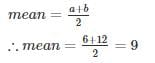Test: Engineering Mathematics- 9 - Question 4

Consider a discrete random variable Y that takes values -2, -1, 0, 1, and 2 with probability 0.2 each. The values of the cumulative distribution function G(y) at y = -1 and y = 1 are _____ and _____ respectively.

Detailed Solution for Test: Engineering Mathematics- 9 - Question 4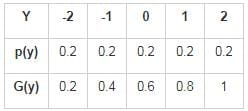G(-1) = p(-2) + p(-1)

= 0.2 + 0.2 = 0.4

G(1) = p(-2) + p(-1) + p(0) + p(1)

= 0.2 + 0.2 + 0.2 + 0.2

= 0.8

Test: Engineering Mathematics- 9 - Question 5

A die is tossed thrice. Success is getting 2 or 5 on a toss. What is the variance of the number of success?

Detailed Solution for Test: Engineering Mathematics- 9 - Question 5

P(success) = Probability of getting 2 or 5 is 2/6 = 1/3

P(failure) = Probability of not getting 2 or 5 is 4/6 = 2/3
Probability of 0 success and 3 failure is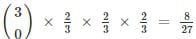Probability of 1 success and 2 failure is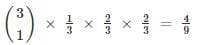Probability of 2 success and 1 failure is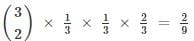Probability of 3 success and 0 failure is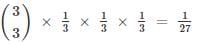x is the number of success and p(x) is probability of x times success.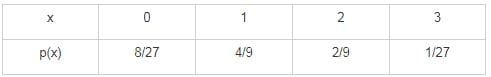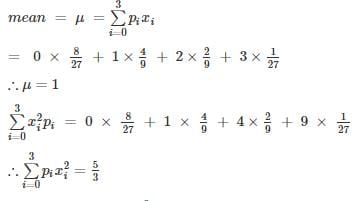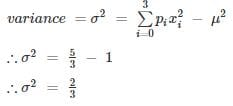Test: Engineering Mathematics- 9 - Question 6

What is the variance of the random variable X whose probability mass function is given below?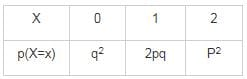Detailed Solution for Test: Engineering Mathematics- 9 - Question 6

q2 + 2pq + p2 = 1

(p+q)2 = 1

∴ (p+q) = 1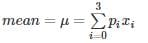= 0 × q2 + 1 × 2pq + 2 × p2

= 2p (q+p)

∴ μ = 2p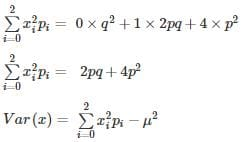Var(x) = 2pq + 4p2 − 4p2

Var(x) = 2pq

Test: Engineering Mathematics- 9 - Question 7

3, k, 2, 8, m, 3

The arithmetic mean of the list of numbers above is 4. If k and m are integers and k ≠ m, what is the median of the list?

Detailed Solution for Test: Engineering Mathematics- 9 - Question 7

Mean = 4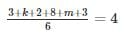k ≠ m so k = m = 4 is out.

{k, m} = {1, 7} or {2, 6} or {3, 5}

for median of {3, k, 2, 8, m, 3}

{1, 2, 3, 3, 7, 8} or {2, 2, 3, 3, 6, 8} or {2, 3, 3, 3, 5, 8}

Median: (3 + 3) /2 = 3

*Answer can only contain numeric values
Test: Engineering Mathematics- 9 - Question 8

If a random variable X has a Poisson distribution with variance 6, then expectation [E(X + 3)2] equals _____.

Detailed Solution for Test: Engineering Mathematics- 9 - Question 8

Var(X) = E(X) = 6

Var(X) = E(X2) - (E(X))2

6 = E(X2) – 36

∴ E(X2) = 42

[E(X + 3)2]

= E(X2 + 6X + 9 )

= E(X2) + 6E(X) + 9

= 42 + 6× 6 + 9

= 87

Test: Engineering Mathematics- 9 - Question 9

The probability density function of a random variable function y has the following probability function: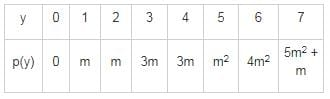find p(y ≥ 5)?

Detailed Solution for Test: Engineering Mathematics- 9 - Question 9

Since y is a random variable: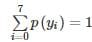= 0 + m+ m + 3m + 3m + m2 + 4m2 + 5m2 + m = 1

10m2 + 9m−1 = 0

(m+1) (10m−1) = 0

∴ m = −1or m = 1/10
since probability cannot be in negative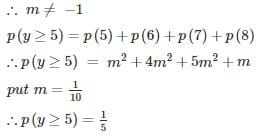Test: Engineering Mathematics- 9 - Question 10

In a binomial distribution, the mean is 4 and variance is 3. Then the mode is

Detailed Solution for Test: Engineering Mathematics- 9 - Question 10

np = 4 and npq = 3

thus q = (3/4) and p = (1-q) = (1/4)

mode is an integer such that np+p > x > np-q

⇒ 4 +(1/4) > x >4-(3/4)

⇒ (13/4)<x<(17/4)

3.25<x<4.25

⇒ x = 4

## RRB JE for Computer Science Engineering

3 videos|7 docs|100 tests
 Use Code STAYHOME200 and get INR 200 additional OFF Use Coupon Code
Information about Test: Engineering Mathematics- 9 Page
In this test you can find the Exam questions for Test: Engineering Mathematics- 9 solved & explained in the simplest way possible. Besides giving Questions and answers for Test: Engineering Mathematics- 9, EduRev gives you an ample number of Online tests for practice

## RRB JE for Computer Science Engineering

3 videos|7 docs|100 tests